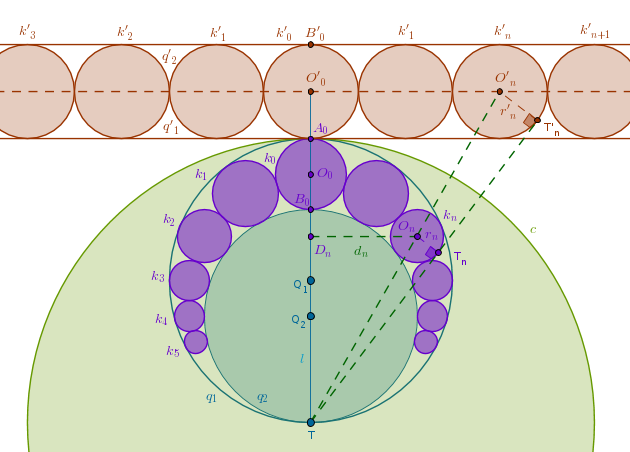Intel's Ronler Acres Plant

# Pergelator

Silicon Forest

## Friday, June 1, 2018

### Tangent CirclesFun with Circles
I came across this bit of geometry on Quora the other day. I haven't quite sorted out just why it works, but if it does, it's pretty cool. I was so impressed with it that I printed a copy and took it lunch to show the gang.

Funny Fractions and Ford Circles - Numberphile

Dennis responds with this video, which also has a bunch of tangent circles along with some goofball math. I saw this and thought that it wouldn't be too much trouble to write a program to verify what's going on here, so I did. Turns out there were a couple of tricky bits that needed sorting, but I think I have it. The first tricky bit was figuring out how much memory I would need. I wrote about this a couple of days ago.

int gcf(int m, int n)    // greatest common factor
{
if ((m==0) || (n==0))
{
if ((m==0) && (n==0)) return 1;
if (m==0) return n;
return m;
}

while(m!=n)
{
if(m > n)
m -= n;
else
n -= m;
}
return m;
}

The next was figuring out how to find the greatest common factor (GCF) of two integers. I've run into this problem before, but where oh where has that bit of code gone? I dunno, but Google finds an example, but it doesn't work. I have to spend several minutes monkeying with it to get it to behave.

That was enough to verify that the Farey addition of fractions works. That is, you generate all of the fractions between zero and one using all denominators from 1 to whatever. Now take any three adjacent fractions on the number line. Add the numerators of the first and last and you will get the numerator of the middle one. Do the same for the denominator and you get the denominator of the middle fraction. You might have to reduce the fraction to make it identical, but the value will be the same regardless.

Verifying that you could use these fractions to generate tangent circles took a little more doing. One way to do it would be to check this out for every new denominator, since at that point the fractions on either side would be the ones you would be forming tangents with. I didn't want to do that, mostly because I was already generating all of the fractions prior to checking the Farey addition, so I need some way to keep track of a fractions "parents" even after multiple fractions had been interposed between them. What I finally settled on was, after generating all fractions for the next denominator, I recorded the values of the parent fractions. Then later I would use these values to locate the original fraction and verify that the generated circles would indeed be tangent.

Tangent Circles and Pythagoras

Verifying that the circles are actually tangent to each other is done by comparing the sum of their radii with the distance between their centers. If these two values are equal they are tangent. If the distance is larger, they are not touching. If the distance is smaller, they overlap.

The distance between centers can be calculated using the Pythagorean Theorem. All you need is the horizontal distance, which is simply the difference between the values of the two fractions, and the vertical difference, which is the difference in their radii. See the above illustration. The orange and purple lines form the sides of the right triangle and the black line forms the hypotenuse.

I fired up my program around 12 hours ago. I gave it some big number to work with, like a 100,000 or something. It has generated over 20 million fractions and it is still running. It seems to be marching on regardless of whether the desktop goes to sleep, or if I am using the computer. I am debating whether I should cancel it or let it keep running. If I remembered what the number was that I gave it, I could estimate how long it is going to run, but I just typed in a one and bunch of zeros. I suppose I should let it run just to make sure it doesn't crash before it finishes.

I've uploaded the source to github if you are interested. I intend to clean up the output so it gives a better picture of what it's doing. When I have done that I will update github.